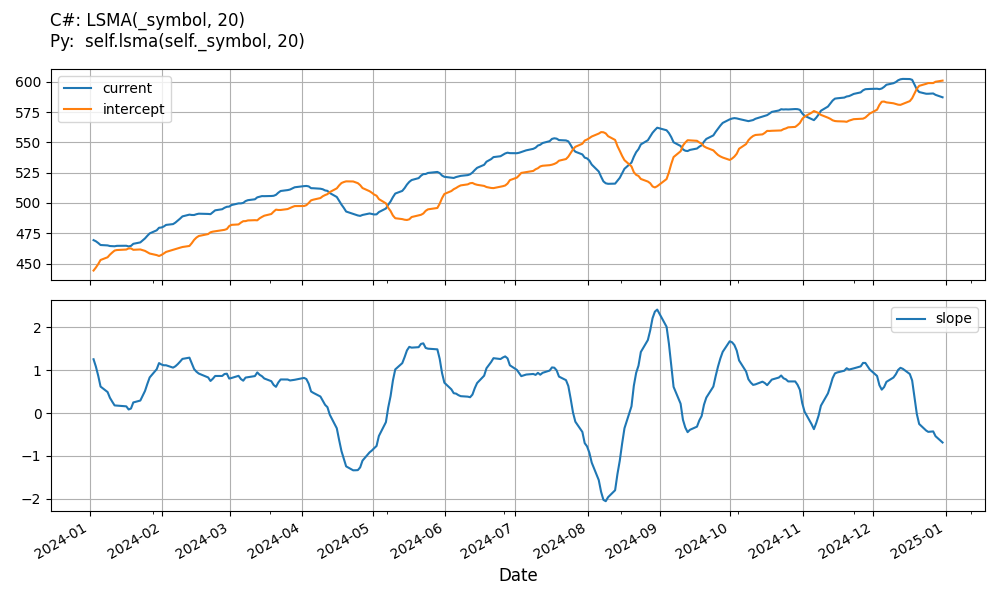# Supported Indicators

## Least Squares Moving Average

### Introduction

The Least Squares Moving Average (LSMA) first calculates a least squares regression line over the preceding time periods, and then projects it forward to the current period. In essence, it calculates what the value would be if the regression line continued. source

To view the implementation of this indicator, see the LEAN GitHub repository.

### Using LSMA Indicator

To create an automatic indicators for LeastSquaresMovingAverage, call the LSMA helper method from the QCAlgorithm class. The LSMA method creates a LeastSquaresMovingAverage object, hooks it up for automatic updates, and returns it so you can used it in your algorithm. In most cases, you should call the helper method in the Initialize method.

public class LeastSquaresMovingAverageAlgorithm : QCAlgorithm
{
private Symbol _symbol;
private LeastSquaresMovingAverage _lsma;

public override void Initialize()
{
_lsma = LSMA(_symbol, 20);
}

public override void OnData(Slice data)
{
{
// The current value of _lsma is represented by itself (_lsma)
// or _lsma.Current.Value
Plot("LeastSquaresMovingAverage", "lsma", _lsma);
// Plot all properties of lsma
Plot("LeastSquaresMovingAverage", "intercept", _lsma.Intercept);
Plot("LeastSquaresMovingAverage", "slope", _lsma.Slope);
}
}
}
class LeastSquaresMovingAverageAlgorithm(QCAlgorithm):
def Initialize(self) -> None:
self.lsma = self.LSMA(self.symbol, 20)

def OnData(self, slice: Slice) -> None:
# The current value of self.lsma is represented by self.lsma.Current.Value
self.Plot("LeastSquaresMovingAverage", "lsma", self.lsma.Current.Value)
# Plot all attributes of self.lsma
self.Plot("LeastSquaresMovingAverage", "intercept", self.lsma.Intercept.Current.Value)
self.Plot("LeastSquaresMovingAverage", "slope", self.lsma.Slope.Current.Value)


The following reference table describes the LSMA method:

### LSMA()1/1

            LeastSquaresMovingAverage QuantConnect.Algorithm.QCAlgorithm.LSMA (
Symbol                           symbol,
Int32                            period,
*Nullable<Resolution>      resolution,
*Func<IBaseData, Decimal>  selector
)


Creates and registers a new Least Squares Moving Average instance.

If you don't provide a resolution, it defaults to the security resolution. If you provide a resolution, it must be greater than or equal to the resolution of the security. For instance, if you subscribe to hourly data for a security, you should update its indicator with data that spans 1 hour or longer.

You can manually create a LeastSquaresMovingAverage indicator, so it doesn't automatically update. Manual indicators let you update their values with any data you choose.

Updating your indicator manually enables you to control when the indicator is updated and what data you use to update it. To manually update the indicator, call the Update method with time/number pair, or an IndicatorDataPoint. The indicator will only be ready after you prime it with enough data.

public class LeastSquaresMovingAverageAlgorithm : QCAlgorithm
{
private Symbol _symbol;
private LeastSquaresMovingAverage _lsma;

public override void Initialize()
{
_lsma = new LeastSquaresMovingAverage(20);
}

public override void OnData(Slice data)
{
if (data.Bars.TryGeValue(_symbol, out var bar))
{
_lsma.Update(bar.EndTime, bar.Close);
}

{
// The current value of _lsma is represented by itself (_lsma)
// or _lsma.Current.Value
Plot("LeastSquaresMovingAverage", "lsma", _lsma);
// Plot all properties of lsma
Plot("LeastSquaresMovingAverage", "intercept", _lsma.Intercept);
Plot("LeastSquaresMovingAverage", "slope", _lsma.Slope);
}
}
}
class LeastSquaresMovingAverageAlgorithm(QCAlgorithm):
def Initialize(self) -> None:
self.lsma = LeastSquaresMovingAverage(20)

def OnData(self, slice: Slice) -> None:
bar = slice.Bars.get(self.symbol)
if bar:
self.lsma.Update(bar.EndTime, bar.Close)

# The current value of self.lsma is represented by self.lsma.Current.Value
self.Plot("LeastSquaresMovingAverage", "lsma", self.lsma.Current.Value)
# Plot all attributes of self.lsma
self.Plot("LeastSquaresMovingAverage", "intercept", self.lsma.Intercept.Current.Value)
self.Plot("LeastSquaresMovingAverage", "slope", self.lsma.Slope.Current.Value)


To register a manual indicator for automatic updates with the security data, call the RegisterIndicator method.

public class LeastSquaresMovingAverageAlgorithm : QCAlgorithm
{
private Symbol _symbol;
private LeastSquaresMovingAverage _lsma;

public override void Initialize()
{
_lsma = new LeastSquaresMovingAverage(20);
RegisterIndicator(_symbol, _lsma, Resolution.Daily);
}

public override void OnData(Slice data)
{
{
// The current value of _lsma is represented by itself (_lsma)
// or _lsma.Current.Value
Plot("LeastSquaresMovingAverage", "lsma", _lsma);
// Plot all properties of lsma
Plot("LeastSquaresMovingAverage", "intercept", _lsma.Intercept);
Plot("LeastSquaresMovingAverage", "slope", _lsma.Slope);
}
}
}
class LeastSquaresMovingAverageAlgorithm(QCAlgorithm):
def Initialize(self) -> None:
self.lsma = LeastSquaresMovingAverage(20)
self.RegisterIndicator(self.symbol, self.lsma, Resolution.Daily)

def OnData(self, slice: Slice) -> None:
# The current value of self.lsma is represented by self.lsma.Current.Value
self.Plot("LeastSquaresMovingAverage", "lsma", self.lsma.Current.Value)
# Plot all attributes of self.lsma
self.Plot("LeastSquaresMovingAverage", "intercept", self.lsma.Intercept.Current.Value)
self.Plot("LeastSquaresMovingAverage", "slope", self.lsma.Slope.Current.Value)


The following reference table describes the LeastSquaresMovingAverage constructor:

### LeastSquaresMovingAverage()1/2

            LeastSquaresMovingAverage QuantConnect.Indicators.LeastSquaresMovingAverage (
string  name,
int     period
)


Initializes a new instance of the LeastSquaresMovingAverage class.

### LeastSquaresMovingAverage()2/2

            LeastSquaresMovingAverage QuantConnect.Indicators.LeastSquaresMovingAverage (
int  period
)


Initializes a new instance of the LeastSquaresMovingAverage class.

### Visualization

The following image shows plot values of selected properties of LeastSquaresMovingAverage using the plotly library.You can also see our Videos. You can also get in touch with us via Discord.